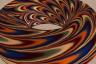http://people.csail.mit.edu/jaffer/Marbling/Torus

# Marbling the Torus

The entire R×R plane is affected by comb homeomorphisms. How would we marble some other two-dimensional manifold, or create a marbled wallpaper design which matches itself at the edges?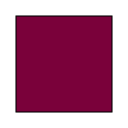### The Torus

Imagine a rectangular sheet rolled so that one edge meets its opposite edge. In order for a pattern drawn on the sheet to blend invisibly, the colors and boundary-line slopes must match at the joined edges.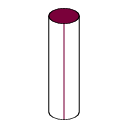Now imagine that tube stretched and rolled so that its top and bottom circles meet. As before, to look continuous the colors and boundary lines must match.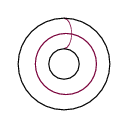This is the torus. To a 2-dimensional observer in the torus surface, the universe looks infinite, but repetitive; looking directly north or south multiple copies of each feature are evenly spaced; looking directly east or west the copies of each feature are evenly spaced -- but not necessarily with the same spacing as appears north-south.

### Flatland

If we unbend the torus sheet and tile the plane with it (all with the same orientation), the 2-dimensional perception of the universe is unchanged; features repeat at uniform intervals both horizontally and vertically. The seams between copies of the sheet will be invisible. Thus the creation of a marbled torus and marbling wallpaper are equivalent.

Many of the deformations discussed so far are not well-defined on the torus.

• A straight tilted tine-line can wrap through the rectangular sheet multiple times depending on its slope and the rectangle's repeat dimensions.

• Large-diameter circles will be self intersecting, which invalidates their treatment in chapter 1.

• Determining the interior of contours looks to be complicated; it may be beyond PostScript's abilities in practice.

Since each feature appears infinitely many times, the total displacement will be an infinite sum. A given point always lies in one half-open interval between a straight stroke and one of its (parallel) images. Without loss of generality, assume that a point at (x,y) lies to the right of a vertical stroke (at xL). The sum of displacements due to the stroke and its images (spaced by rx) to the left side is:
 ∑ j z·ux−xL+j·rx = z·ux−xL ∞ ∑ j=0 (urx)j = z·ux−xL 1−urx
The sum of displacements due to the right-side images is:
 ∑ j z·urx+xL−x+j·rx = z·urx+xL−x ∞ ∑ j=0 (urx)j = z·urx+xL−x 1−urx
The total displacement is then:
 z ux−xL + urx+xL−x 1 − urx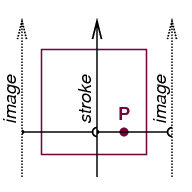Because all strokes will be subject to the constant 1/(1−urx) deepening factor, we don't bother to compute it and let the artist control it through z. Thus we need sum only the contributions from the two closest lines. Because the contributions are symmetrical to both sides of the stroke, it doesn't matter which side of the interval is half-open when the point lies on the stroke.

### Execution

Creating homeomorphisms from the toroid patch onto itself requires discipline:
• The period of a sinusoid (or other) parallel displacement must be an integer harmonic of the period of the whole image rectangle in that direction.

• The wraparound of coordinates is accomplished by calling fmod of any coordinate which changes in the Composite-map sequence.

```/fmod 	% real modulus --> real
{
/X2 exch def /X1 exch def
X1 X2 X1 X2 div floor mul sub
} bind def
```
• The new stroke function determines which side of its stroke the incoming point is. In addition to calculating the displacement due to the primary stroke, it adds in the displacement due to the closest image.

Because the repeat lengths xrep and yrep may be different, I returned to using separate routines for horizontal and vertical strokes.

```/Vertical	% px py xc dy --> px py
{   /dy exch def
/xc exch def
/py exch def /px exch def
/a  xc px sub abs u exch exp z mul
px xc lt {xc xrep sub} {xc xrep add} ifelse
px sub abs u exch exp z mul add def
px py dy a mul add yrep fmod
} bind def

/Horizontal	% px py yc dx --> px py
{   /dx exch def
/yc exch def
/py exch def /px exch def
/a  yc py sub abs u exch exp z mul
py yc lt {yc yrep sub} {yc yrep add} ifelse
py sub abs u exch exp z mul add def
px dx a mul add xrep fmod py
} bind def

/Up {-1 Vertical} bind def
/Down {1 Vertical} bind def
/Left {1 Horizontal} bind def
/Right {-1 Horizontal} bind def
```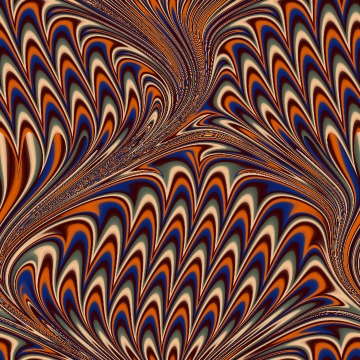The resulting (oversampled) bouquet image can tile the plane with perfect continuity across seams. A monochrome version of this pattern is the background for these pages.

[image is linked to bouquet tiling]

The images below are from an earlier version of mathematical marbling (hyperbolic vs. exponential); I can't find a VRML viewer which does image mapping (2010-12).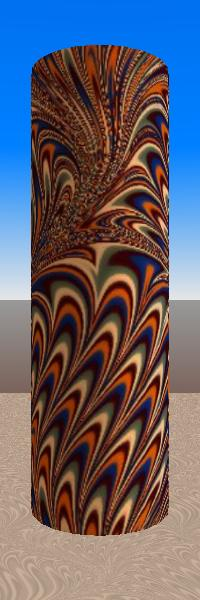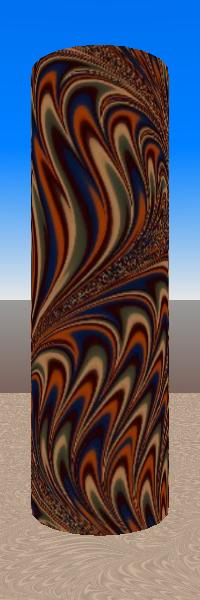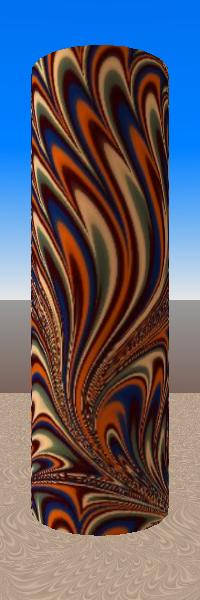The next chapter explores application for these designs.